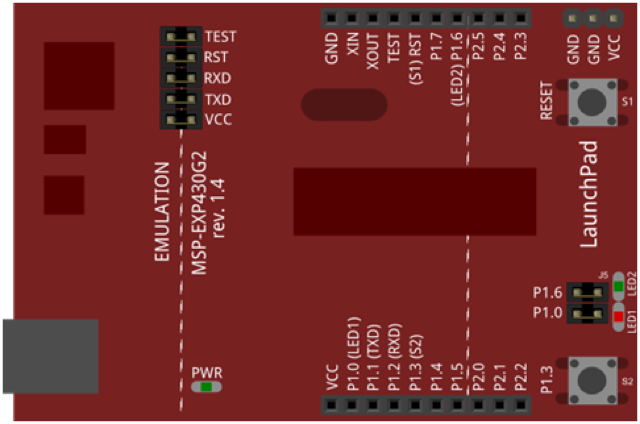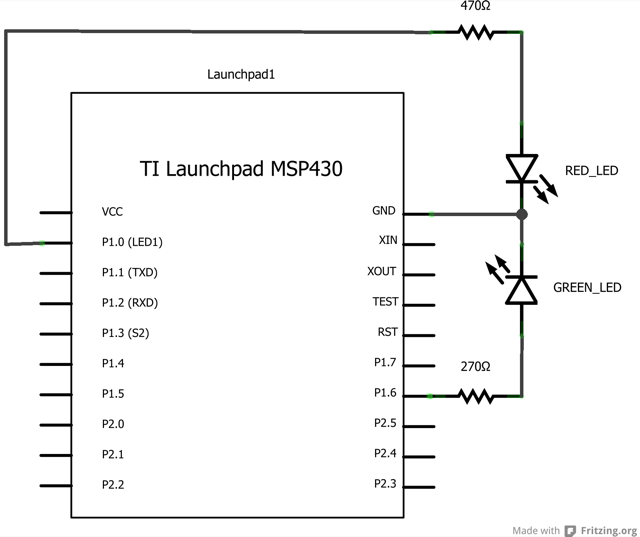Demonstrates the advanced serial printing functions by generating a table of characters and their ASCII values in decimal, hexadecimal, octal, and binary. For more on ASCII, see asciitable.com

None

### CircuitImage developed using Fritzing. For more circuit examples, see the Fritzing project page.

### Schematic### Code Explanation

This code demonstrates the serial printing functions that can be performed, using Energia, by generating a table of characters and their ASCII values in decimal, hexadecimal, octal, and binary. `Serial.write()` function outputs the ASCII symbol associated with the number. By associating the variable thisByte, we can start at the beginning of the ASCII Table (33 which corresponds to 33 in decimal base and increase till we reach 126 representing the tilde (~) symbol. `Serial.print()` allows you print directly to the Serial Monitor in different base formats depending on your need. Using these two printing functions we can display the entire ASCII Table in our Serial Monitor.

### Code

```/*
ASCII table

Prints out byte values in all possible formats:
* as raw binary values
* as ASCII-encoded decimal, hex, octal, and binary values

For more on ASCII, see http://www.asciitable.com and http://en.wikipedia.org/wiki/ASCII

created 2006
by Nicholas Zambetti
modified 9 Apr 2012
by Tom Igoe
modified 25 April 2013

This example code is in the public domain.

*/
void setup() {
//Initialize serial and wait for port to open:
Serial.begin(9600);

// prints title with ending line break
Serial.println("ASCII Table ~ Character Map");
}

// first visible ASCIIcharacter '!' is number 33:
int thisByte = 33;
// you can also write ASCII characters in single quotes.
// for example. '!' is the same as 33, so you could also use this:
//int thisByte = '!';

void loop() {
// prints value unaltered, i.e. the raw binary version of the
// byte. The serial monitor interprets all bytes as
// ASCII, so 33, the first number, will show up as '!'

Serial.write(thisByte);

Serial.print(", dec: ");
// prints value as string as an ASCII-encoded decimal (base 10).
// Decimal is the default format for Serial.print() and Serial.println(),
// so no modifier is needed:
Serial.print(thisByte);
// But you can declare the modifier for decimal if you want to.
//this also works if you uncomment it:

// Serial.print(thisByte, DEC);

Serial.print(", hex: ");
// prints value as string in hexadecimal (base 16):
Serial.print(thisByte, HEX);

Serial.print(", oct: ");
// prints value as string in octal (base 8);
Serial.print(thisByte, OCT);

Serial.print(", bin: ");
// prints value as string in binary (base 2)
// also prints ending line break:
Serial.println(thisByte, BIN);

// if printed last visible character '~' or 126, stop:
if(thisByte == 126) { // you could also use if (thisByte == '~') {
// This loop loops forever and does nothing
while(true) {
continue;
}
}
// go on to the next character
thisByte++;
}```

### Try it out

• Play around with your RGB LED in a new way.